Fluid Mechanics Practice Level -1

# Fluid Mechanics Practice Level -1

Test Description

## 40 Questions MCQ Test | Fluid Mechanics Practice Level -1

Fluid Mechanics Practice Level -1 for Class 11 2022 is part of Class 11 preparation. The Fluid Mechanics Practice Level -1 questions and answers have been prepared according to the Class 11 exam syllabus.The Fluid Mechanics Practice Level -1 MCQs are made for Class 11 2022 Exam. Find important definitions, questions, notes, meanings, examples, exercises, MCQs and online tests for Fluid Mechanics Practice Level -1 below.
Solutions of Fluid Mechanics Practice Level -1 questions in English are available as part of our course for Class 11 & Fluid Mechanics Practice Level -1 solutions in Hindi for Class 11 course. Download more important topics, notes, lectures and mock test series for Class 11 Exam by signing up for free. Attempt Fluid Mechanics Practice Level -1 | 40 questions in 80 minutes | Mock test for Class 11 preparation | Free important questions MCQ to study for Class 11 Exam | Download free PDF with solutions
 1 Crore+ students have signed up on EduRev. Have you?
Fluid Mechanics Practice Level -1 - Question 1

### A solid uniform ball of volume V floats on the interfaceof two immiscible liquids (see the figure). The specificgravity of the upper liquid is 1 r and that of lower oneis 2 r and the specific gravity of ball is 1 2 r (r < r < r ) .The fraction of the volume of the ball in the upper liquid is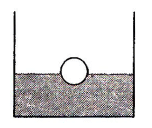Fluid Mechanics Practice Level -1 - Question 2

### A tank is filled up to a height 2H with a liquid and isplaced on a platform of height H from the ground. Thedistance x from the ground where a small hole ispunched to get the maximum R isFluid Mechanics Practice Level -1 - Question 3

### In the figure, the cross-sectional area of teh smallertube is a and that of the larger tube is 2a. A block ofmass m is kept in the smaller tube having the samebase area a, as that of the tube. The difference betweenwater levels of the two tubes is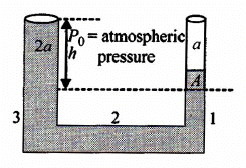Fluid Mechanics Practice Level -1 - Question 4

An ornament weighing 36 g in air weights only 34 g inwater. Assuming that some copper is mixed with goldto prepare the ornament, find the amount of copper init. Specific garvity of gold is 19.3 and that of copper is8.9

Fluid Mechanics Practice Level -1 - Question 5

Water flows through a horizontal tube as shown in thefigure. If the difference of heights of water column in thevertical tubes is h = 0.02 m. and the area of crosssection at A and B are 4 × 10-4 m2 and 2 × 10-4 m2,respectively, then the rate of flow of water across anysection is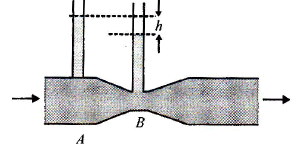Fluid Mechanics Practice Level -1 - Question 6

In a U-tube in which the cross-sectional area of thelimb on the left is one-quater, the limb on the containsmercury (density 13.6 g/cm3), and the level of mercuryin the narrow limb is at a distance of 36 cm from theupper end of the tube. What will be the rise in the levelof mercury in the right limb if the left limb is filled to thetop with water?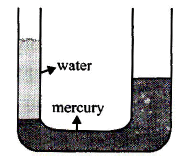Fluid Mechanics Practice Level -1 - Question 7

We have two different liquids A and B whose relative iensities are 0.75 and 1.0, respectively. If we dip solid objects P and Q having relative densities 0.6 and 0.9 in these liquids, then

Fluid Mechanics Practice Level -1 - Question 8

A cubical block of wood of side a and density ρ floats in water of density 2 ρ . The lower surface of the cube just touches the free end of a massless spring of force constant K fixed at the bottom of the vessel. The weight W put over the block so that it is completely immersed
in water without wetting the weight is

Fluid Mechanics Practice Level -1 - Question 9

When at rest, a liquid stands at the same level in the‘tubes as shown in the figure. But as indicated, a heightdifference h occurs when the system is given anacceleration 'a' towards the right. Then h is equal to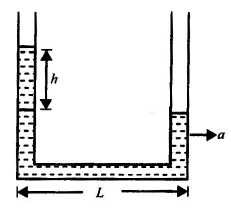Fluid Mechanics Practice Level -1 - Question 10

Three liquids having densities 1 2 ρ ρ and 3 ρ are filled ina U-tube. Length of each liquid column is equal to I,1 2 3 ρ > ρ > ρ liquids remain at rest (relative to thetube) in the position shown in the figure. It is possiblethat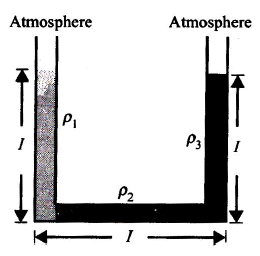Fluid Mechanics Practice Level -1 - Question 11

A beaker containing water is placed on the platform of a spring balance. The balance reads 1.5 kg. A stone of mass 0.5 kg and density 104 kg/m3 is immersed in water without touching the walls of the beaker. What will be the balance reading now?

Fluid Mechanics Practice Level -1 - Question 12

“figure shows a capillary tube C dipped in a liquid thatwets The liquid rises to a point A. If we blow air throughthe horizontal tube H, what will happen to the liquidcolumn in the capillary tube?

Fluid Mechanics Practice Level -1 - Question 13

A tank is filled with water of density 103 kg/m3 and oilof density 9 X 103 kg/m3. The height of water layer is 1m and that of the oil layer is 4 m. The velocity of effluxfrom an opening in the bottom of the tank is

Fluid Mechanics Practice Level -1 - Question 14

A hole is made at the bottom of a tank filled with water(density = 103 kg/m3). If the total pressure at the bottomof the tank is 3 atm (1 atm = 105 N/m2), then the velocityof efflux is

Fluid Mechanics Practice Level -1 - Question 15

The opening near the bottom of the vessel shown infigure has an area A. A disc is held against the openingto keep the liquid from running out. Let F1 be the netforce on the disc applied by liquid and air in this case.Now the disc is moved away from the opening a shortdistance. The liquid comes out and strikes the discinelastically. Let F2 be the force exerted by the liquid inthis condition. Then F1 / F2 is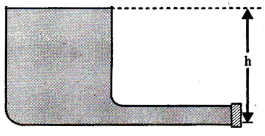Fluid Mechanics Practice Level -1 - Question 16

There are two identical small holes of area of crosssection a on the opposite sides of a tank containing aliquid ‘of density ρ . The difference in height betweenthe holes is h. The tank is resting on a smoothhorizontal surface. The horizontal force which will haveto be applied on the tank to keep it in equilibrium is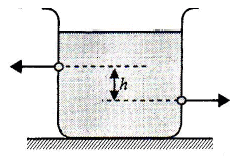Fluid Mechanics Practice Level -1 - Question 17

Equal volume of two immiscible liquids of densities rand are 2 ρ filled in a vessel as shown in the figure.Two small holes are punched at depths h/2 and 3h/2from the surface of lighter liquid. If v1 and v2 are thevelocities of efflux at these two holes, then v1/v2 is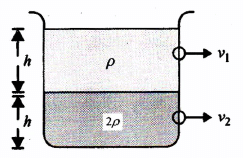Fluid Mechanics Practice Level -1 - Question 18

In a cylindrical water tank there are two small holes Qand P on the wall at a depth of h1, from the upper levelof water and at a height of h2 from the lower end of thetank, respectively, as shown in the figure. Water comingout from both the holes strike the ground at the samepoint. The ratio of h1 and h2 is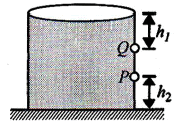Fluid Mechanics Practice Level -1 - Question 19

Figure shows a liquid flowing through a tube at thefate of 0.1 m3/s. The tube is branched into twosemicircular tubes of cross-sectional area A/3 and24/3. The velocity of liquid at Q is (the cross section ofthe main tube is A = 102 m2 and vP = 20 m/s)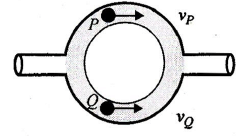Fluid Mechanics Practice Level -1 - Question 20

The tension in a string holding a solid block below thesurface of a liquid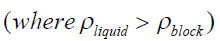as in shownin the figure is T when the system is at rest.Then what will be the tension in the string if the systemhas upward acceleration a?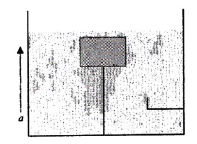Fluid Mechanics Practice Level -1 - Question 21

An ideal fluid flows in the pipe as shown in the figure, pressure in the fluid at the bottom P2 is the same as at the top P1. If the velocity of the top v1 = 2 m/s. Then the ratio of areas A1 / A2 is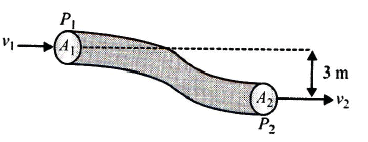Fluid Mechanics Practice Level -1 - Question 22

A sphere of solid material of specific gravity 8 has aconcentric spherical cavity and just sinks in water. Theratio of radius of cavity to that of outer radius of thesphere must be

Fluid Mechanics Practice Level -1 - Question 23

A cylindrical container of radius ‘R' and height ‘h’ iscompletely filled with a liquid. Two horizontal L-shapedpipes of small cross-sectional area ‘a’ are connectedto the cylinder as shown in the figure. Now the twopipes are opened and fluid starts coming out of thepipes horizontally in opposite directions. Then thetorque due to ejected liquid on the system is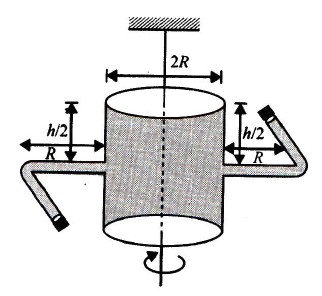Fluid Mechanics Practice Level -1 - Question 24

Figure shows water filled in a symmetrical container,four pistons of equal area A are used at the fouropenings to keep the water in equilibrium. Now anadditional force F is applied at each piston. Theincrease in the pressure at the centre of the containerdue to this addition is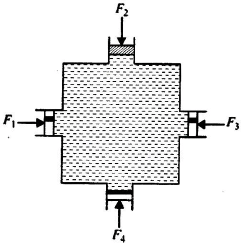Fluid Mechanics Practice Level -1 - Question 25

An open tank 10 m long and 2 m deep is filled up to 1.5 m height of oil of specific gravity 0.82. The tank is uniformly accelerated along its length from rest to a speed of 20 m/s horizontally. The shortest time in which the speed may be attained without spilling any oil is a.

Fluid Mechanics Practice Level -1 - Question 26

A U-tube of base length 'l ' filled with the same volumeof two liquids of densities ρ and 2 ρ is moving with anacceleration ‘a’ on the horizontal plane. If the heightdifference between the two surfaces (open toatmosphere) becomes zero, then the height h is givenby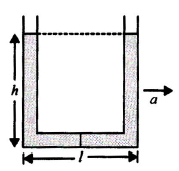Fluid Mechanics Practice Level -1 - Question 27

A narrow tube completely filled with a liquid is lying ona series of cylinders as shown in the figure. Assumingno sliding between any surfaces, the value ofacceleration of the cylinders for which liquid will notcome out of the tube from anywhere is given by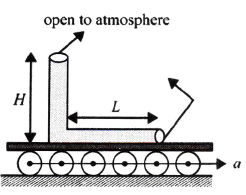Fluid Mechanics Practice Level -1 - Question 28

The velocity of the liquid coming out of a small hole ofvessel containing two different liquids of densities 2rani r as shown in the figure is

Fluid Mechanics Practice Level -1 - Question 29

A non-uniform cylinder of mass m, length / and radius ρ is having its centre of mass at a distance 1/4 fromthe centre and lying on the axis of the cylinder. Thecylinder is kept in a liquid of uniform density ρ . Themoment of inertia of the rod about the centre of massis I. The angular acceleration of point A relative to pointB just after the rod is released from the position asshown in the figure is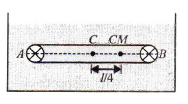Fluid Mechanics Practice Level -1 - Question 30

A square box of water has a small hole located in one of the bottom corners. When the box is full and sitting on a level surface, complete opening of the hole results in a flow of water with a speed v0, as shown in Fig. (a). When the box is still half empty, it is tilted by 45° so that the hole is at the lowest point. Now the water will flow out with a speed of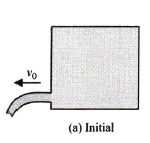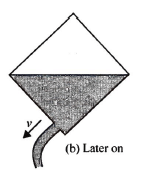Fluid Mechanics Practice Level -1 - Question 31

A cylindrical block is floating (part-ially submerged) ina vessel containing water. Initially, the platform on whichthe vessel is mounted is at rest. Now the platform alongwith the vessel is allowed to fall freely under gravity. Asa result, the buoyancy force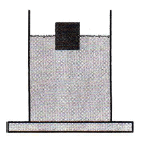Fluid Mechanics Practice Level -1 - Question 32

For the L-shaped vessel shown in the figure, determinethe value of acceleration a so that pressure at point Abecomes equal to p0/2? (p0 is the atmospheric pressure)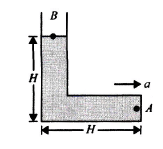Fluid Mechanics Practice Level -1 - Question 33

An empty balloon weighs W1. If air equal in weight to W is pumped into the balloon, the weight of the balloon becomes W2. Suppose that the density of air inside and outside the balloon is the same. Then

Fluid Mechanics Practice Level -1 - Question 34

A tube 1 cm2 in cross section is attached to the top ofa vessel 1 cm high and of cross section 100 cm2. Wateris poured into the system filling it to a depth of 100 cmabove the bottom of the vessel as shown in the figure.Take g = 10 ms-2. Find the correct statement.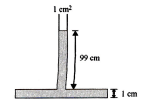Fluid Mechanics Practice Level -1 - Question 35

A smaIl ball of density ρ is immersed in a liquid ofdensity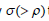to a depth h and released. The heightabove the surface of water up to which the ball willjump is

Fluid Mechanics Practice Level -1 - Question 36

A cylindrical tank of height H is open at the top end andit has a radius r. Water is filled in it up to a height of h.The time taken to empty the tank through a hole ofradius r' in its bottom is

Fluid Mechanics Practice Level -1 - Question 37

A square of size 1 m × 1 m is hinged at its midpoint. Afluid of density ρ fills the space to the left of the gate.The force F required to hold the gas stationary is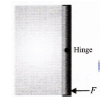Fluid Mechanics Practice Level -1 - Question 38

Equal masses of three liquids are kept in three identialcylindrical vessels A,B and C. Their densitiesare , ρA ρB  and ρC with PA < PB < PC.The force on thebase will be

Fluid Mechanics Practice Level -1 - Question 39

A body B is capable of remaining stationary inside a liquid at the position shown in Fig (a). If the whole system is gently placed on smooth inclined plane (Fig b.) and is allowed to slide down, then (0 < θ < 900 )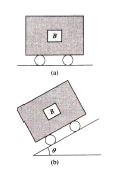Fluid Mechanics Practice Level -1 - Question 40

A sealed tank containing a liquid of density ρ moveswith horizontal acceleration a as shown in the figure.The difference in pressure between two points A and Bwill be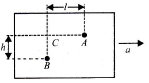Use Code STAYHOME200 and get INR 200 additional OFF Use Coupon Code
Information about Fluid Mechanics Practice Level -1 Page
In this test you can find the Exam questions for Fluid Mechanics Practice Level -1 solved & explained in the simplest way possible. Besides giving Questions and answers for Fluid Mechanics Practice Level -1, EduRev gives you an ample number of Online tests for practice